Question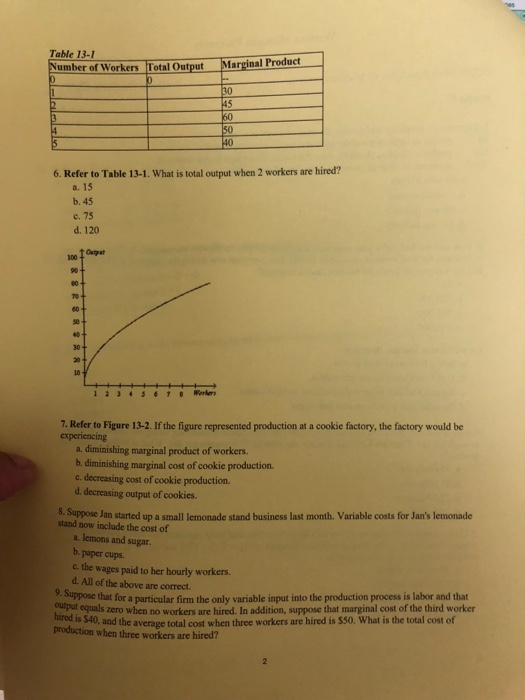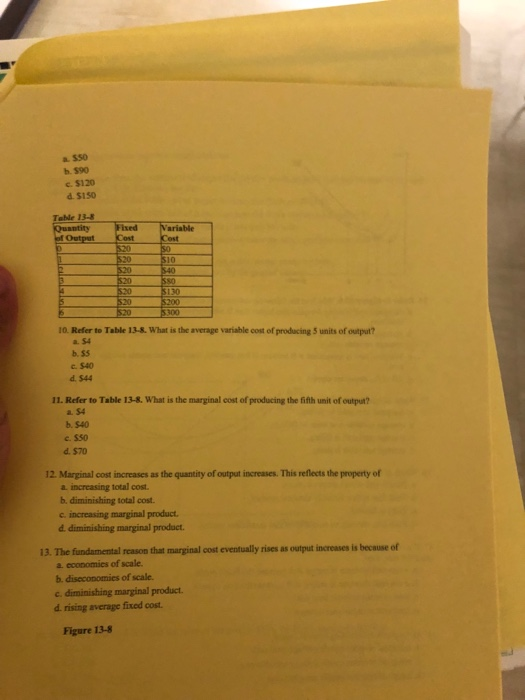Reason

Total output with one worker= 0+30= 30

Total output with two worker= 30+45=75

Answer 7. A. Diminishing marginal product of labor

Reason

Marginal product is the slope of production function. When number of workers increases by 1 unit, the marginal product rises by a small amount.

Answer 8. All of the above.

Reason

Wages paid, paper cups, sugar lemonade are all the factors that vary with the amount of lemonade produced. So they are variable costs.

Reason

Total cost when 3 workers are hired= ATC*3 = \$50*3= \$150

#### Earn Coins

Coins can be redeemed for fabulous gifts.

Similar Homework Help Questions
• ### Question 6 S L 1 pts Table 13-5 Number of Output Workers 0 0 1 1 ,000 2 2 ,000 S32,700 4 3 ,200 5 3 ,500 6 3 ,600...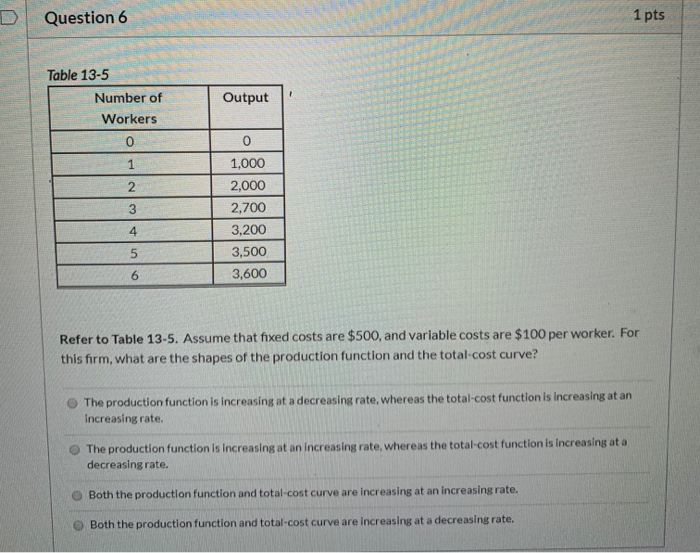Question 6 S L 1 pts Table 13-5 Number of Output Workers 0 0 1 1 ,000 2 2 ,000 S32,700 4 3 ,200 5 3 ,500 6 3 ,600 Refer to Table 13-5. Assume that fixed costs are \$500, and variable costs are \$100 per worker. For this firm, what are the shapes of the production function and the total cost curve? The production function is increasing at a decreasing rate, whereas the total cost function is increasing at...

• ### Question 17 (1 point) Table 13-1 Number of Workers Total Output Marginal Product x Refer to...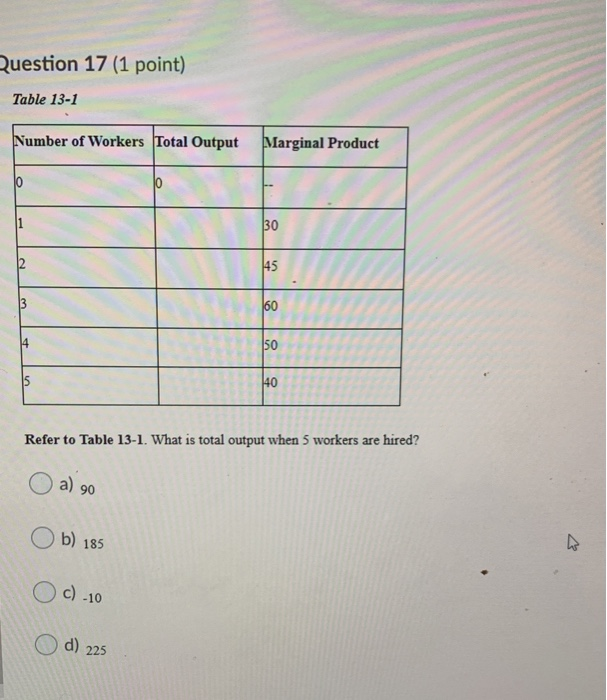Question 17 (1 point) Table 13-1 Number of Workers Total Output Marginal Product x Refer to Table 13-1. What is total output when 5 workers are hired? O b) 185 O O c) 10 O O O d) 225

• ### Table 13-1 Number of Workers Total Output Marginal Product 0 0 -- 1 30 2 40...

Table 13-1 Number of Workers Total Output Marginal Product 0 0 -- 1 30 2 40 3 50 4 40 5 30 Refer to Table 13-1. What is total output when 5 workers are hired? Group of answer choices 160 -10 190 120

• ### Number of Total Marginal Workers Output Product 300 500 600 650 4 1) Refer to the...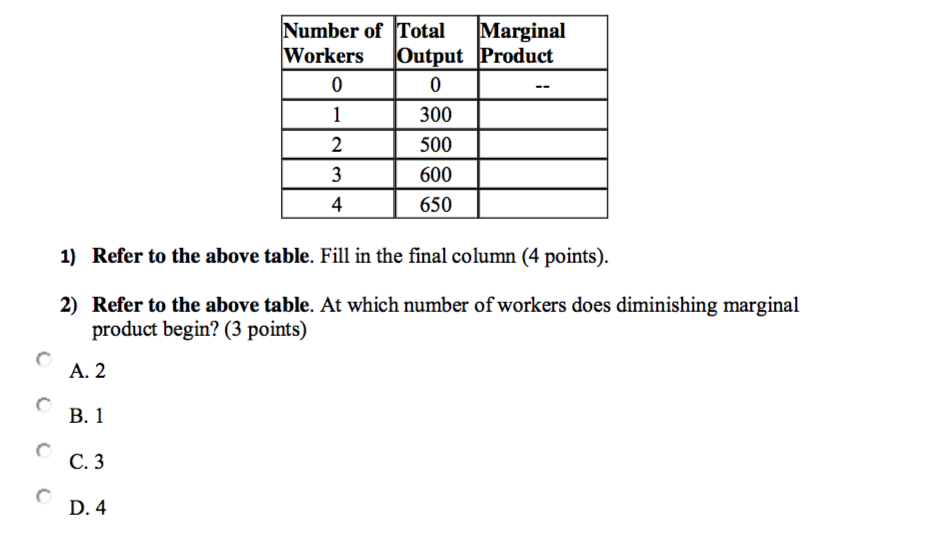Number of Total Marginal Workers Output Product 300 500 600 650 4 1) Refer to the above table. Fill in the final column (4 points). 2) Refer to the above table. At which number of workers does diminishing marginal product begin? (3 points) A. 2 B. 1 C. 3 D. 4

• ### e total cost 19. For a certain firm, the 10th unit of output marginal cost of...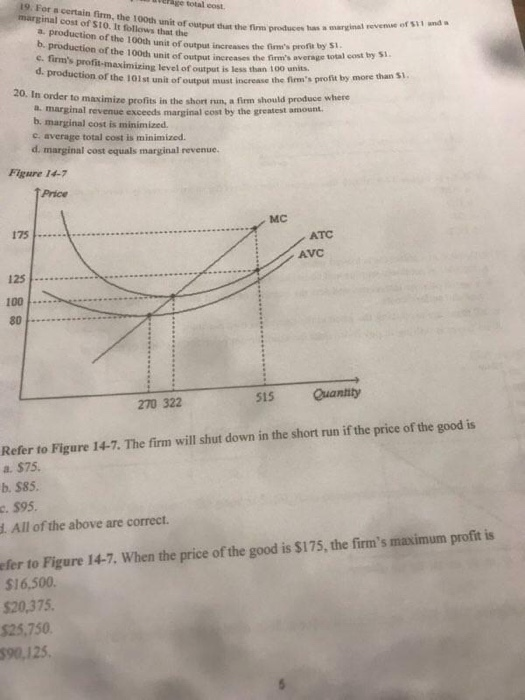e total cost 19. For a certain firm, the 10th unit of output marginal cost of Sto. It follows that the production of the 10th it fo r of outputut the firm produse marinat revenue of land them the firm's profi not the 100th unit of t h e firm's average total costs C. Firm's profit-maximize ve futut is less than 100 units. d. production of the 101st unit of output the lost unit of output must increase the firm's...

• ### Table 13-2 Number of Total Marginal Workers Output Product 300 500 600 1650 Refer to Table...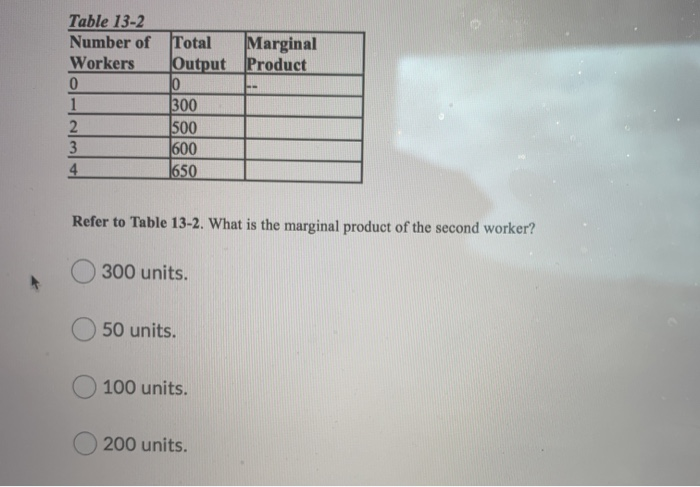Table 13-2 Number of Total Marginal Workers Output Product 300 500 600 1650 Refer to Table 13-2. What is the marginal product of the second worker? O 300 units. 50 units. 100 units. 200 units.

• ### Table 13-7 2 Number of Workers Total Output 0 Marginal Product 40 85 125 35 30 215 Refer to Table 13-7. What is the marginal product of the third worker? a. 45 O b. 125 О с. 40 O d. 85 Table 13-...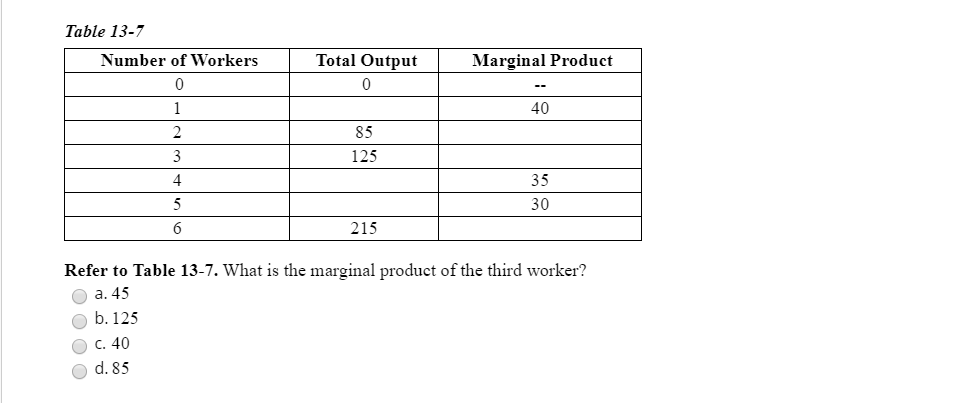Table 13-7 2 Number of Workers Total Output 0 Marginal Product 40 85 125 35 30 215 Refer to Table 13-7. What is the marginal product of the third worker? a. 45 O b. 125 О с. 40 O d. 85 Table 13-7 2 Number of Workers Total Output 0 Marginal Product 40 85 125 35 30 215 Refer to Table 13-7. What is the marginal product of the third worker? a. 45 O b. 125 О с. 40 O...

• ### 17. Refer to Table 13-2. At which number of workers does diminishing marginal product begin? ooo...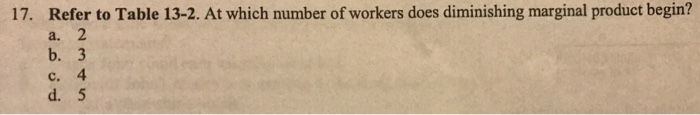17. Refer to Table 13-2. At which number of workers does diminishing marginal product begin? ooo Table 13-2 Number of Workers Output 0 1 2 3 50 110 180 260 330 14. Assume a certain firm regards the number of workers it employs as variable, and that it regards the size of its factory as fixed. This assumption is often realistic a. in the short run, but not in the long run. b. in the long run, but not in...

• ### Cabinets question Diminishing marginal returns occur when: O total product decreases. O each additional unit of...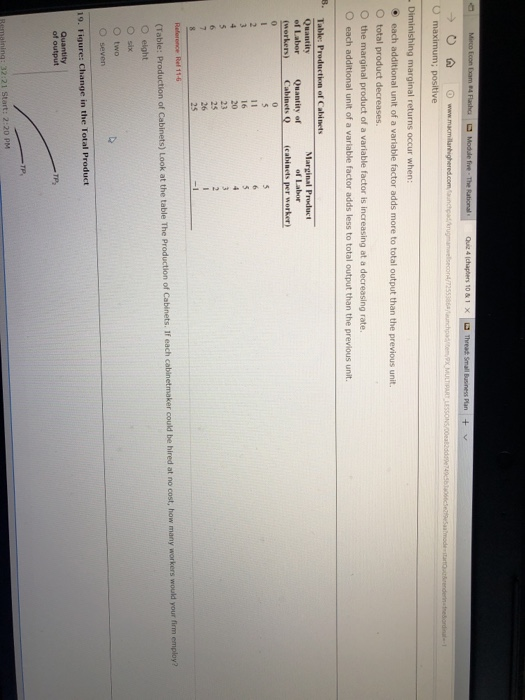Cabinets question Diminishing marginal returns occur when: O total product decreases. O each additional unit of a variable factor adds more to total output than the previous unit. the marginal product of a variable factor is increasing at a decreasing rate. each additional unit of a variable factor adds less to total output than the previous unit. Marginal Product of Labor of Labor Quantity of (workers) Cabinets (cabinets per worker) cost, how many workers would your firm employ? (Table: Production...

• ### For a production function with a diminishing, but positive, marginal product of labor: A. Output increases...

For a production function with a diminishing, but positive, marginal product of labor: A. Output increases at an increasing rate as more workers are employed B. Output increases at a decreasing rate as more workers are employed. C. Output declines as more workers are employed. D. The effects on marginal product are unknown.